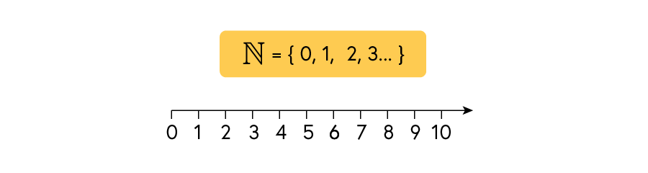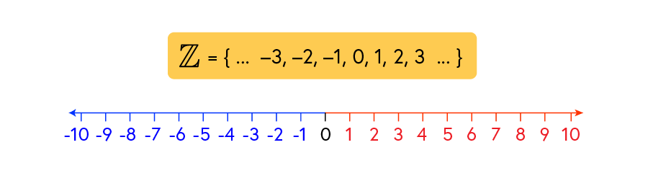## Want to keep learning?

This content is taken from the National STEM Learning Centre's online course, Maths Subject Knowledge: Understanding Numbers. Join the course to learn more.
3.2

# Negative numbers

We started the course by looking at the counting numbers. With the inclusion of zero, the counting numbers are known as the Natural Numbers.With the advent of money and the purchasing of goods, came the idea of borrowing money from a money lender, and debt. To express this concept, negative numbers were developed. The Natural number line is extended to the left to include all whole numbers whether they be positive or negative.

This is called the set of integers.The set of integers are denoted by the letter Z which comes from the German word ‘Zahlen’ which means ‘number’.

Instead of counting up from zero towards infinity we can just as simply set off counting backwards along our line. From 0 to -1 to -2 to negative infinity. In addition to the multiples of 2 in the positive direction, we can also think about the multiples of negative 2, by taking two steps along in the negative direction.

Have you ever considered what the negative two times table looks like?

We can think of the negative part of our number line as a mirror image of the positive part of the number line; two opposite, equal parts that make up the number line, often referred to as ‘directed numbers’.

It is important to note that “negative three” and “minus three” mean two different things. Negative three is a number, whereas the minus three is an operation: take away three. For clarity is useful to make this distinction clear when working with students. In the next steps, we will explore how to add, subtract, multiply and divide negative numbers.

## Discussion

Students are usually confident that 5 is greater than 3. What would you do to correct the misconception that if 5 is greater than 3 then -5 is greater than -3?